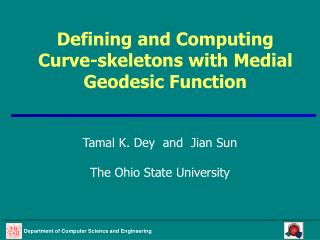# Defining and Computing Curve-skeletons with Medial Geodesic Function - PowerPoint PPT PresentationDownload PresentationDefining and Computing Curve-skeletons with Medial Geodesic Function

Defining and Computing Curve-skeletons with Medial Geodesic FunctionDownload Presentation## Defining and Computing Curve-skeletons with Medial Geodesic Function

- - - - - - - - - - - - - - - - - - - - - - - - - - - E N D - - - - - - - - - - - - - - - - - - - - - - - - - - -
##### Presentation Transcript

1. Defining and Computing Curve-skeletons with Medial Geodesic Function Tamal K. Dey and Jian Sun The Ohio State University

2. Motivation • 1D representation of 3D shapes, called curve-skeleton, useful in many application • Geometric modeling, computer vision, data analysis, etc • Reduce dimensionality • Build simpler algorithms • Desirable properties[Cornea et al. 05] • centered, preserving topology, stable, etc • Issues • No formal definition enjoying most of the desirable properties • Existing algorithms often application specific

3. Contributions • Give a mathematical definition of curve-skeletons for 3D objects bounded by connected compact surfaces • Enjoy most of the desirable properties • Give an approximation algorithm to extract such curve-skeletons • Practically plausible

4. Roadmap

5. Medial axis • Medial axis: set of centers of maximal inscribed balls • The stratified structure [Giblin-Kimia04]: generically, the medial axis of a surface consists of five types of points based on the number of tangential contacts. • M2: inscribed ball with two contacts, form sheets • M3: inscribed ball with three contacts, form curves • Others:

6. Medial geodesic function (MGF)

7. Properties of MGF • Property 1 (proved): f is continuous everywhere and smooth almost everywhere. The singularity of f has measure zero in M2. • Property 2 (observed): There is no local minimum of f in M2. • Property 3 (observed): At each singular point x of f there are more than one shortest geodesic paths between ax and bx.

8. Defining curve-skeletons • Sk2=SkÅM2: set of singular points of MGF or points with negative divergence w.r.t. rf • Sk3=SkÅM3: extending the view of divergence • A point of other three types is on the curve-skeleton if it is the limit point of Sk2[ Sk3 • Sk=Cl(Sk2[ Sk3)

9. Computing curve-skeletons • MA approximation [Dey-Zhao03]: subset of Voronoi facets • MGF approximation: f(F) and (F) • Marking: E is marked if (F)²n <  for all incident Voronoi facets • Erosion: proceed in collapsing manner and guided by MGF

10. Examples

11. Properties of curve-skeletons • Thin (1D curve) • Centered • Homotopy equivalent • Junction detective • Stable Prop1: set of singular points of MGF is of measure zero in M2 Medial axis is in the middle of a shape Prop3: more than one shortest geodesic paths between its contact points Medial axis homotopy equivalent to the original shape Curve-skeleton homotopy equivalent to the medial axis

12. Effect of 

13. Shape eccentricity and computing tubular regions • Eccentricity: e(E)=g(E) / c(E)

14. Thank you!